# RTRA SAS Program Guide

This guide helps users get started with writing a SAS program/code for RTRA purposes.

As described on the StatCan webpage, "the RTRA system is an on-line remote access facility allowing users to run SAS programs, in real-time, against microdata sets located in a central and secure location". Statistics Canada provides multiple avenues to access data and statistics: StatCAN census profile tables, CANSIM, RTRA and RDC. RTRA is appropriate for researchers looking for aggregate data that is not available through PUMFs (Public Use Microdata Files) but have too little time or too small a project to request access to the RDC.

### RESOURCES

The following resources are useful to begin writing your SAS program.

• Identifying a survey of interest:
• Identifying variables of interest:
• Questionnaire(s) for survey of interest
• Dummy survey dataset (FTP resource)
• Survey dataset codebook (FTP resource)
• Writing the SAS code:

### WRITING THE SAS PROGRAM

You can write a SAS program from scratch or modify the survey specific sample SAS code provided on the FTP server (usually called SampleProgramEng.sas found under the Example Program folder of the survey directory). Not all surveys provide a sample SAS code.

#### OVERALL STEPS

1. Library step to define a short term to represent the data directory (libname)
• If you have a libname line in your SAS code delete it. If you don’t, ignore this step.
2. Data step to process the master dataset
• The survey master dataset is called from the RTRAData library/directory
• The name of this master dataset can be found on the RTRA Parameters webpage
3. Macro step to compute statistics
4. Name the SAS program file starting with the TAG name specific to the survey followed by an underscore
• The TAG name can be found on the RTRA Parameters webpage
• Eg. CCHS2015_myname

Note: The data step is necessary even if you are not modifying the original dataset because you cannot refer to the RTRAData library in the macro step.

#### BASIC CODE STRUCTURE

The RTRA SAS code consists of 1) the data step and 2) the procedure macro step. The data step is used to process data by keeping/dropping observations and/or variables, creating new variables etc. The procedure macro step are used to compute means, frequencies, percentiles, percent distributions, proportions, ratios and shares.

The list of procedure macro steps are:

• %RTRAFreq
• %RTRAMean
• %RTRAPercentile
• %RTRAPercentDist
• %RTRAProportion
• %RTRARatio
• %RTRAShare

#### DATA STEP

The SAS code for the data step has the following structure below. Each line (statement) ends with a semi-colon. The final statement run is used to execute previous lines of SAS statements. The asterisks are meant to be replaced with the appropriate parameter names.

```data ******;
****** ******;
run;
```

Below are the definitions of the parameters to be replaced.

```data ******;       -> name of the processed data set. Use one term with no spaces.
****** ******;     -> add statements to process data
run;
```

Additional statements in the data step are optional and only to be included if you want to process the master dataset before running a procedure. These statements are included between the set line and the run line. The lines to be added depend on how you want to modify the original survey dataset. A few examples of additional statements that can be included in the data step can be found below.

To drop observations (i.e., subset) use the if statement

##### Eg. Drop all values of gender with a value of 1
`     if gender=1 then delete;`
##### Eg 2. To delete cases where the variable education has missing values [dot=missing for numeric]
`     if education = . then delete;`

To create a new variable which results in a new column

##### Eg. Create a new variable days that converts the variable year from the original data set
`     days = 365.25*year;`
##### Eg 2. To convert a character variable age with a length of 3 digits into a numeric variable age2
`     age2 = input(age, 3);`

To recode a variable

##### Eg. Say a variable maritalstatus has values 5 that we want to recode (regroup) as 4
`     if maritalstatus = 5 then maritalstatus = 4 ;`

Additional documentation on SAS statements used in the data step can be found here and here.

Some numeric measures are stored as character variables. To run procedures such as the mean procedure on variables stored as character, you have to convert them to numeric first. If you are not sure if the variable is stored as numeric or character, you can either assume it is numeric and run the procedure and to see if you get an error message or you can check the survey documentation found on the FTP server.

#### MACRO STEP - FREQUENCY & MEAN PROCEDURE

The SAS code for the frequency and mean macro procedures hav the following structure below. Again, the asterisks are meant to be replaced with the appropriate names. The definiton of arguments follows after the SAS code for each procedure. The SAS code/list of arguments for other procedures can be found here. Note that not all arguments are always necessary when running a procedure. For example, the ClassVarList variable may not be necessary depending on the output desired and the procedure used. When this is the case, simply omit the ClassListVar argument (the entire line) from the SAS code.

```%RTRAFreq(
InputDataset =******,
OutputName=******,
ClassVarlist=******,
UserWeight=****** );
```

Definition of arguments (Freq)

InputDataset: Add the data set used to run procedure (the processed data set)
OutputName: Name the output data table (the results of the procedure in a data table)
ClassVarlist: Add variable(s) used to make the frequency table
UserWeight: Add survey weight variable found in the Parameters document

```%RTRAMean(
InputDataset =******,
OutputName=******,
ClassVarlist=******,
AnalysisVarList=******,
UserWeight=****** );
```

Definition of arguments (Mean)

InputDataset: Add the data set used to run procedure (the processed data set)
OutputName: Name the output data table (the results of the procedure in a data table)
ClassVarlist: Add variable(s) used to group mean calculation
AnalysisVarList: Add variable(s) used to calculate mean
UserWeight: Add survey weight variable found in the Parameters document

#### EXAMPLE OF FINAL SAS CODE

Below is an example of an RTRA SAS code.

```data ******;
****** ******;
run;
```
```%RTRAFreq(
InputDataset =******,
OutputName=******,
ClassVarlist=******,
UserWeight=****** );
```
```%RTRAMean(
InputDataset =******,
OutputName=******,
ClassVarlist=******,
AnalysisVarList=******,
UserWeight=****** );
```

### MACROS

RTRAFreq gives the weighted count of observations at each level of the Class variable(s). This procedure is appropriate for discrete variables because a Class variable cannot have more than 500 distinct values – as per the RTRA system limitation. The first row of the output table is the total weighted count without breaking it down by the Class variable. With every additional variables, a new variable column is added and a count for each combination of levels is given. The image below shows the output tables from the frequency procedure using one Class variable (left) and two Class variables (right).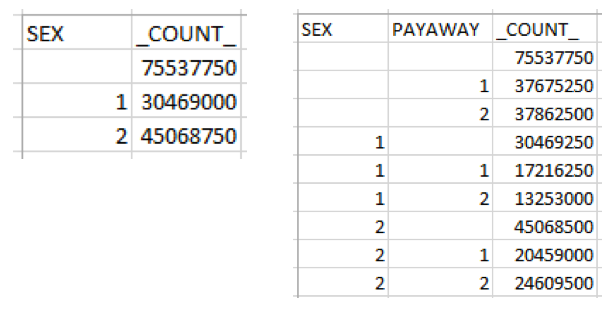RTRAPercentDist gives the relative frequency or percentage of observations at each level or combination of levels of the Class variable(s). When more than one Class variable is specified, this procedure is equivalent to cell and marginal percentages in a 2x2 frequency table. The weighted count is also provided.

RTRAProportion gives, within each respective category of the Class variable(s), the percentage of observations at each level of the ByVar variable. The Class variable is the grouping variable. This procedure is equivalent to row and/or column percentages in a 2x2 frequency table. The weighted count is also provided.

The image below shows the output tables from the PercentDist procedure (left) and the Proportion procedure (right).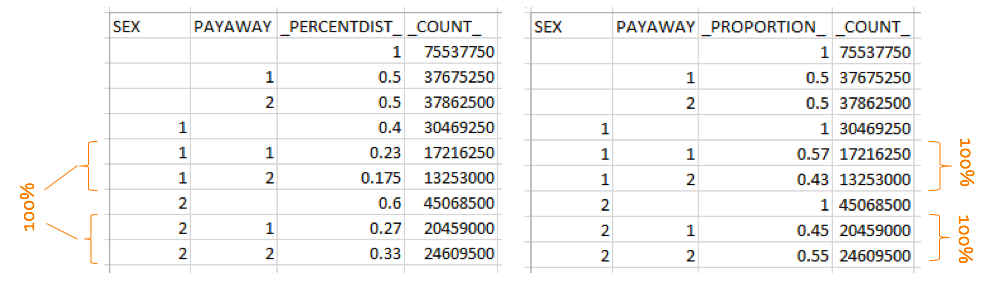RTRAMean provides the mean or what is commonly known as the average of the AnalysisVarList variable at each level of the Class variable.  If more than one Class variable is specified, it gives the mean of the AnalysisVarList variable at each combination of levels of the Class variables. The weighted count is also provided.

RTRAPercentile gives the value of the AnalysisVar variable below which a certain percent of observations fall (i.e., the Nth percentile of the AnalysisVar variable) for each level of the Class variable.  You cannot run this procedure without a Class variable. You can choose 3 percentiles to calcualte from the following list: 1, 5, 10, 20, 25, 30, 40, 50, 60, 70, 75, 80, 90, 95, 99. The weighted count is also provided.

The image below shows the output tables from the Mean procedure (left) and the Percentile procedure (right).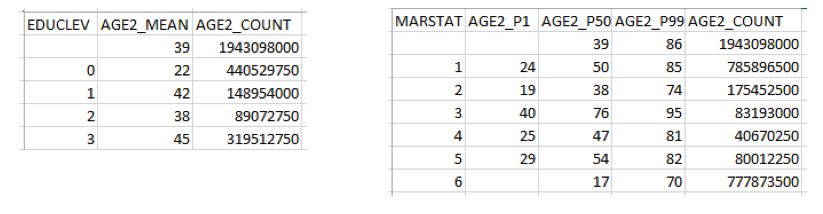RTRARatio gives the ratio of the totals of two continuous variables (NumeratorVar and DenominatorVar) at each level of the ByVar variable. When a Class variable is added, the ratio is given for each combination of the Class and ByVar variables. The weighted count is also provided.

RTRAShare gives a percentage which is the subtotal (sum) of the Share variable at each level of the ByVar variable divided by the grand total of the Share variable: the share of the Share variable at each level of the ByVar variable. When a Class variable is added, the shares are calculated at each level of the Class variable. The shares add up to 100% within each level of the Class variable. The weighted count is also provided.

Higher-order statistics can be calculated using modified versions of these procedures. You can learn more about such procedures here.

### SUBMIT THE SAS PROGRAM

Once you have completed your SAS program, you want to submit it!

2. Click on To StatCan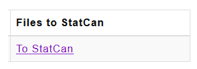### RTRA OUTPUT

It takes a few minutes to run the code. You will likely receive an email when the output is ready.

2. Click on From StatCan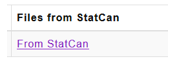The output files will be available for 7 days before they are deleted. The files are ordered by RTRA code number. Use the time of submission to find the most recent output files.

List of output files:

• RTRA log file (.txt) indicates which stages of the RTRA processes have been successfully completed.
• SAS log file (.log)
• Output table as a SAS data set, CSV file and HTML file

The statistics reported in the output tables are rounded to the nearest rounding base. The rounding base for each survey can be found in the RTRA parameters webpage.

You will find rows in the output tables with blank cell(s). The statsitics in a row with a blank cell is not broken down by the variable in the blank cell column. In other words, the statistics in that specific row is reported as if that variable was not included in the analysis.

#### ERROR

It is not uncommon to get errors when you first submit your program. Use the checklist below to check and debug your SAS program.

Tips/Checklist:

• Check the RTRA log file if the RTRA process was unsuccesful at any stage
• Check the SAS log file for error messages
• Use the survey tag name at the beginning of the SAS program file name (eg. CCHS2015_zzzz)
• The library name is always RTRAData
• Do not include a libname line
• Use the dataset name in the data step following the RTRAData library name
• Don’t forget the semi-colon after every line in the data step!
• Don’t forget the semi-colon at the end of the macro!
• Don’t forget run; at the end of the data step
• Check the spelling of variables, procedure(s), data set name
• Check the RTRA system limitations